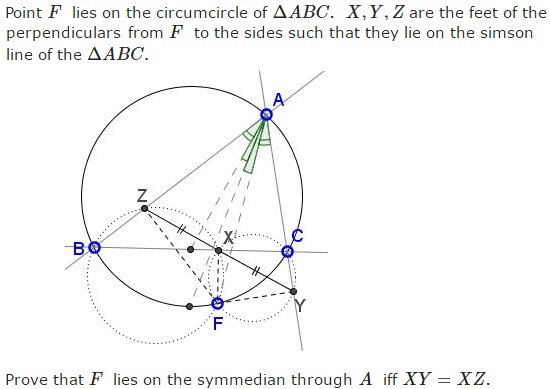# Symmedian and the Simson

### Problem### Proof 1

We first assume that $F\;$ lies on the symmedian from $A\;$ and shall repeatedly apply the Law of Sines.

In $\Delta FXZ,\;$ $\displaystyle\frac{XZ}{FX}=\frac{\sin\angle XFZ}{\sin\angle FZX}\;$ in $\Delta XYF,\;$ $\displaystyle\frac{XY}{FX}=\frac{\sin\angle XFY}{\sin\angle FYX}.\;$ Eliminating $FX$ gives

(1)

$\displaystyle\frac{XZ}{XY}=\frac{\sin\angle XFZ}{\sin\angle XFY}\cdot\frac{\sin\angle FYX}{\sin\angle FZX}.$In $\Delta FYZ,\;$ $\displaystyle \frac{\sin\angle FYX}{\sin\angle FZX}=\frac{\sin\angle FYZ}{\sin\angle FZY}=\frac{FZ}{FY}.\;$ Since $F\;$ lies on the symmedian, it follows that $\displaystyle \frac{FZ}{FY}=\frac{AB}{AC}.$

On the other hand, quadrilateral $BFXZ\;$ is cyclic so that $\angle XFZ=\angle XBZ =\angle ABC.\;$ Similarly, $\angle XFY=\angle ACB.\;$ In $\Delta ABC,\;$ $\displaystyle\frac{\sin\angle XFZ}{\sin\angle XFY}=\frac{\sin\angle ABC}{\sin\angle ACB}=\frac{AC}{AB}.$

Substituting our finds into (1) shows that

$\displaystyle\frac{XZ}{XY}=\frac{AB}{AC}\cdot\frac{AC}{AB}=1.$

implying $XY=XZ.$

Conversely, assume $XY=XZ.$ Then (1) becomes,

$\displaystyle 1=\frac{\sin\angle XFZ}{\sin\angle XFY}\cdot\frac{\sin\angle FYX}{\sin\angle FZX}=\frac{AC}{AB}\cdot\frac{FZ}{FY},$

implying $\displaystyle\frac{FY}{FZ}=\frac{AC}{AB},\;$ which is a characteristic property of points on the symmedian.

### Proof 2

Let $E\;$ be the point on the circle where $EF\parallel BC,\;$ $F'\;$ be the point of intersection of $AE\;$ and $FX.\;$ We make the following observations:1. It immediately follows that $AF\;$ and $AE\;$ isogonal conjugates of triangles $BAC\;$ and $ZAY\;$ too. Since $AF\;$ is diameter of circumcircle $ZAY\;$ (quadrilateral $AZFY\;$ is cyclic) it follows that $AE\;$ perpendicular to the Simson line $XYZ,\;$ i.e., $F'\;$ is the intersection of the altitudes of $\Delta ZAY.$

2. In $\Delta ZAY,\;$ point $X\;$ on side $ZY\;$ bisects $FF'\;$ if and only if $F'\;$ is the orthocenter of that triangle, in other words, $FX=F'X\;$ is equivalent to $ZX=XY\;$ $(FZFY\;$ must be a parallelogram.)

3. If we look now at right triangle $EFF'\;$ we'll see that $FX=F'X\;$ iff $M_a\;$ is on the hypotenuse $EF'\;$ as the circumcenter of that triangles $(AE\;$ is a median).

Thus we arrive at the following conclusions:

1. Line $AE\;$ is perpendicular to the simson line of point $F\;$ iff $AE,\;$ $AF\;$ are isogonal conjugates.

2. $ZX=XY\;$ is equivalent to $AM_a\;$ being perpendicular to $XYZ.$

### Proof 3

The condition $ZX=XY\;$ can be rewritten as: $BF\cdot\sin (B) = CF\cdot\sin(C)\;$ which is equivalent to

$\displaystyle\frac{AC}{BC}=\frac{\sin(B)}{\sin(C)} = \frac{\sin(CAF)}{\sin(BAF)}=\frac{FY}{FZ}.$

But this is one of the characteristic properties of the symmedian.

### Acknowledgment

1. The statement and Proof 2 are due to Vladimir Nikolin who posted both at the CutTheKnotMath facebook page. Vladimir also posted a proof (Proof 3) from the artofproblemsolving site.### Symmedian